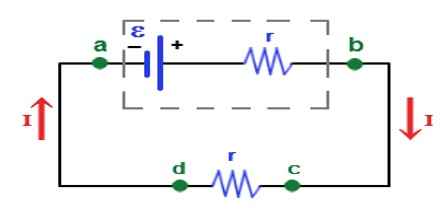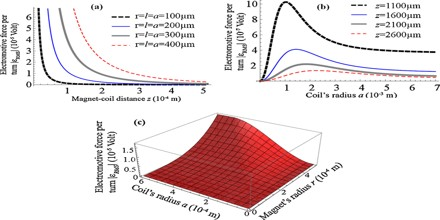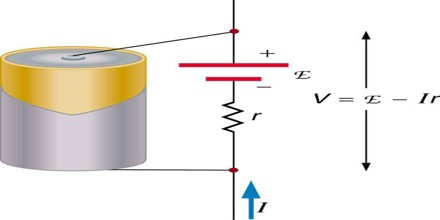EMF: Electromotive Force

Definition

Electromotive Force or EMF is a source of energy that can cause a current to flow in an electrical circuit or device. A device that supplies electrical energy is called Electromotive Force or EMF. EMFs convert chemical, mechanical, and other forms of energy into electrical energy. The product of such a device is also known as EMF. The word “Force” in this case is not used to mean mechanical force, measured in newtons, but a potential, or energy per unit of charge, measured in volts.Electromotive Force (EMF) is not actually a force; it is commonly measured in units of volts, equivalent in the metre–kilogram–second system to one joule per coulomb of electric charge. In the electrostatic units of the centimetre–gram–second system, the unit of electromotive force is the statvolt, or one erg per electrostatic unit of charge.

EMF is the external work expended per unit of charge to produce an electric potential difference across two open-circuited terminals. The electric potential difference produced is created by separating positive and negative charges, thereby generating an electric field. The created electrical potential difference drives current flow if a circuit is attached to the source of emf. When current flows, however, the voltage across the terminals of the source of emf is no longer the open-circuit value, due to voltage drops inside the device due to its internal resistance.

Around 1830, Michael Faraday established that the reactions at each of the two electrode–electrolyte interfaces provide the “seat of emf” for the voltaic cell, which is, these reactions drive the current and are not an endless source of energy as was initially thought. In the open-circuit case, charge separation continues until the electrical field from the separated charges is sufficient to arrest the reactions. Years earlier, Alessandro Volta, who had measured a contact potential difference at the metal–metal (electrode–electrode) interface of his cells, had held the incorrect opinion that contact alone, without taking into account a chemical reaction was the origin of the emf.Function of Electromotive Force (EMF)

An electromotive force is the potential difference developed by a voltage source, and is necessary to cause current to flow through a circuit. Strictly-speaking, it is the open-circuit potential difference of a battery, generator, etc. An alternative definition is that an e.m.f. is equal to the sum of the voltage drops around any closed loop, including any internal voltage drop. The basic function of an emf in a circuit is –

• Convert electrical energy into some other form.
• Convert some other form of energyinto electrical.
• Both choices (a) and (b) are valid.
• None of the above choices are valid.

Difference between Electromotive Force (EMF) and Voltage

An electric generator produces E.M.F inside the generator in the armature which is due to the change of flux in the armature coils and this is a perfect example of E.M.F.

EMF=k*Φ*w …………….where k— a constant, Φ— flux per pole, w—speed of the rotor.

Here is no term of resistance over here. So, emf is generated by a source.

Voltage is just the potential difference between two points in a circuit.

Voltage=I*R ……EMF is the voltage generated by a source like battery or generator.

Voltages in a circuit called ‘voltage drops’ are in the opposite direction of EMF and their sum is equal to EMF according to Kirchhoff’s second law.

Electromotive Force (EMF) is directly related to the source of potential difference, such as the particular combination of chemicals in a battery. However, EMF differs from the voltage output of the device when current flows. The voltage across the terminals of a battery, for example, is less than the emf when the battery supplies current, and it declines further as the battery is depleted or loaded down. However, if the device’s output voltage can be measured without drawing current, then output voltage will equal EMF, even for a very depleted battery.

Actually, the difference between EMF and Voltage is that, voltage is the potential difference in a closed circuit which means when a switch frm a battery or a source is switched on the p.d in the circuit is known as voltage. V=E – Ir. But whereas EMF is the raw potential contained inside a source or battery excluding all the losses. Emf is the max ability of a battery but when in circuit it becomes V taking in the losses occured by the conductor’s resistance. That’s why for extrnl voltage we have the formula V= E – Ir. Whr E is the emf of the cell, I is the total current in the circuit and r is the resistance of the “conductor”. Transformers function on the principle of power, as we know P=VI and I=P/V and V=P/I. Thus I is inversely proportional to V. Thus increase in current results decrease in voltage and vice versa. Transformers have to minimise the loss of current that’s why they transfer with large voltage and small current which they later step up or step down according to requirement.